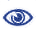# Prague Stringology Conference 2014

Computing Abelian Covers and Abelian Runs

 Abstract: Two strings u and v are said to be Abelian equivalent if u is a permutation of the characters of v. We introduce two new regularities on strings w.r.t. Abelian equivalence, called Abelian covers and Abelian runs, which are generalizations of covers and runs of strings, respectively. We show how to determine in O(n) time whether or not a given string w of length n has an Abelian cover. Also, we show how to compute an O(n2)-size representation of (possibly exponentially many) Abelian covers of w in O(n2) time. Moreover, we present how to compute all Abelian runs in w in O(n2) time, and state that the maximum number of all Abelian runs in a string of length n is (n2).

 Download paper:PostScript PDF BibTeX reference
 Download presentation: